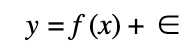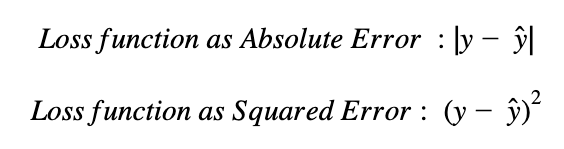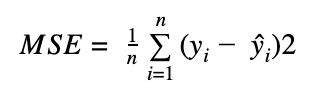# Model Selection in Machine Learning

Model selection refers to choose the best statistical machine learning model for a particular problem. For this task we need to compare the relative performance between models. Therefore the loss function and the metric that represent it, becomes fundamental for selecting the right and non-overfitted model.

We can state a machine learning supervised problem with the following equation:This equation is composed with the x matrix that contains the predictor’s factors x1,x2,x3,…xn. These factors can be the lagged prices/returns of a time series or some others factors such as volume, foreign exchange rates, etc.  y is the response vector that depend of the function f and the predictors x.

f contain the underlying relationship between the x features and the y response and can be modeled with a linear regression if the underlying relationship is linear or with a Random Forest or Support Vector Machine algorithm if the underlying relationship is non-linear.

Ε represent the error term, which is often assumed to have mean zero and a standard deviation of one.

Once we fit a particular model for a certain dataset, we need to define the loss function that we will use to assess model performance. Many measures can be used for the loss function. Some common measures for the loss function are the Absolute Error and the Squared Error between predicted values and real values.Both choices are non-negative, so the best value for the loss function is zero. The Absolute Error and Squared Error above, compute the difference between the true value (y)  and the prediction (y)  for each observation of the dataset.

Both the Absolute Error and Squared Error are vectors or arrays of n x 1 dimension, reflecting the error term per each of the observations. In order to aggregate the error term of a certain model between all the predicted and real values of a variable, a popular measure is the Mean Squared Error which is simply the average of the squared loss:Where n is the number of observations